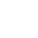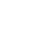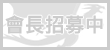LV. 50
GP 8k# 【心得】熱愛的冷人冷作 129 下弦之月 矢澤愛的催淚迷離戀愛漫畫與電影

GP1 BP-

^^^^^^^^^^^^^^^^^^^^^^^^^^^^^^^^^^^^

^^^^^^^^^^^^^^^^^^^^^^^^^^^^^^^^^^^^^^^^^^^^^^^

^^^^^^^^^^^^^^^^^^^^^^^^^^^^

^^^^^^^^^^^^^^^^^^^^^^^^^face基於日前微軟官方表示 Internet Explorer 不再支援新的網路標準，可能無法使用新的應用程式來呈現網站內容，在瀏覽器支援度及網站安全性的雙重考量下，為了讓巴友們有更好的使用體驗，巴哈姆特即將於 2019年9月2日 停止支援 Internet Explorer 瀏覽器的頁面呈現和功能。